Physical Chemistry : Oxidation-Reduction Fundamentals

Example Questions

Example Question #1 : Electrochemistry

Which of the following periodic table groups typically undergo reduction reactions?

Transition metals

Alkaline earth metals

Noble gases

Halogens

Halogens

Explanation:

Reduction reactions are characterized by a gain of electrons whereas oxidation reactions are characterized by a loss of electrons. Recall that elements on the right side of the periodic table have the tendency to gain electrons to complete the octet. Groups such as chalcogens (group VI) and halogens (group VII) have six and seven valence electrons, respectively; therefore, they only need a few electrons to have eight valence electrons and complete the octet. Since halogens tend to gain electrons, they typically undergo reduction.

Alkaline earth metals (group II) and transition metals have few valence electrons; therefore, they tend to lose electrons and undergo oxidation. Noble gases are nonreactive elements and do not undergo reduction or oxidation.

Example Question #1 : Oxidation Reduction Fundamentals

A researcher has two compounds. Compound A is manganese dioxide (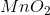) and compound B is potassium permanganate (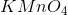). A reaction involving the conversion of manganese in Compound A to manganese in Compound B can be classified as which of the following reactions?

Reduction, because the manganese will gain 3 electrons

Oxidation, because the manganese will lose 3 electrons total

Reduction, because the manganese will lose 3 electrons

Oxidation, because the manganese will gain 3 electrons total

Oxidation, because the manganese will lose 3 electrons total

Explanation:

For this problem, we need to figure out the oxidation states of manganese in each compound. Oxygen typically has an oxidation number ofand potassium has an oxidation number of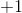. For Compound A, there are two oxygen molecules; therefore, oxygen contributes a total charge of. Since manganese dioxide is a neutral molecule, the manganese in it will have an oxidation number of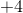. For Compound B, four oxygen atoms contribute a charge ofwhereas one potassium atom contributes a charge of; therefore, the manganese in potassium permanganate will have an oxidation number of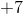. Conversion of manganese from Compound A to manganese in Compound B involves the removal of electrons—three electrons, specifically, because its charge has to change fromto; therefore, this reaction is characterized as an oxidation reaction.

Example Question #1 : Electrochemistry

Which of the following reactions involves both reduction and oxidation reactions?

Single displacement reactions

Both single displacement reactions and the reaction of sodium chloride and calcium sulfate

The reaction of sodium chloride and calcium sulfate

Neither single displacement reactions nor the reaction of sodium chloride and calcium sulfate

Single displacement reactions

Explanation:

A reaction that has both reduction and oxidation half reaction is called a redox reaction. It involves one or more atoms gaining electrons (reduction) and one or more atoms losing electrons (oxidation). Recall that single displacement reactions involve the replacement of an element in a compound with another element. An example of single replacement reaction is shown below: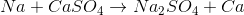In this reaction, a sodium atom replaces a calcium atom in calcium sulfate. If we calculate the oxidation numbers for sodium and calcium, we can see that sodium loses an electron (the oxidation state goes fromto) whereas the calcium ion gains two electrons (goes from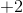to); therefore, this is a redox reaction. All single displacement reactions follow this general trend and are characterized as redox reactions.

Reaction of sodium chloride and calcium sulfate is as follows: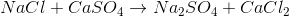If we calculate the oxidation state of each atom we will notice that oxidation number doesn’t change; therefore, this isn’t a redox reaction.

Example Question #2 : Electrochemistry

Oxidation reactions __________ electrons and reduction reactions __________ electrons.

produces . . . produces

consumes . . . produces

consumes . . . consumes

produces . . . consumes